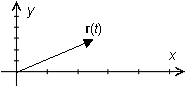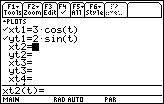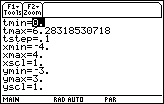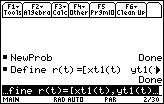Module 24 - Vectors Introduction | Lesson 1 | Lesson 2 | Lesson 3 | Self-Test Lesson 24.2: Vector Functions The functions investigated in pervious modules have been real-valued functions, in which the domain and range are real numbers. In this lesson vector-valued functions, or simply vector functions, will be explored. Defining Vector Functions Vector functions are functions whose domain values are real numbers and whose range consists of vectors. They are often used to describe the motion of an object through space. When a particle moves in a plane during a time interval, its coordinates can be given as parametric functions of time. The parametric equations below can represent the position of a particle at time t. x = f(t) y = g(t) Defining a Position Vector The graph of the parametric equations represents the path of the particle and the vector r(t) = f(t)i + g(t)j begins at the origin and points to the particle's position at time t. Such a vector is called the position vector for the particle at time t. A position vector for time t is illustrated below.The coordinates of the vector are determined by the function values of f and g at time t. Defining the Velocity and Acceleration Vectors As before, the position of a particle can yield the particle's velocity and acceleration. The velocity vector for the particle, v(t), is the derivative of the position vector with respect to time. v(t) = r' (t) = f ' (t)i + g' (t)j The acceleration vector is the second derivative of the position vector with respect to time. a(t) = r" (t) = f " (t)i + g" (t)j Illustrating the Path of a Particle Graph the path of the particle that has position vector r(t) = 3cos(t)i + 2sin(t)j for the time interval [0, 2]. Perform NewProb Select Parametric mode Set xt1(t) = 3 cos(t) Set yt1(t) = 2 sin(t)Use the following viewing windowMake sure your TI-89 is in Radian Mode 24.2.1 Graph the path of the particle. Click here for the answer. Finding the Position Vector Create a function that will return the position vector whose path is defined by xt1 and yt1 in the Parametric mode Y= Editor. Define r(t) = [xt1(t), yt1(t)]24.2.2 Find the position vector for the particle at t =/4 by entering r(/4). Click here for the answer. Finding the Velocity Function Use the derivative command to create a function that will return the velocity vector as a function of time. Define v(t) = d (r(t), t) 24.2.3 Evaluate the velocity vector at t =/4. Click here for the answer. Finding the Acceleration Function Create a function that will return the acceleration vector. Define a(t) = d(r(t), t, 2) 24.2.4 Evaluate the acceleration vector at t =/4. Click here for the answer. Do not clear the functions r, v, and a because they will be used in Lesson 24.3. < Back | Next > ©Copyright 2007 All rights reserved. | Trademarks | Privacy Policy | Link Policy